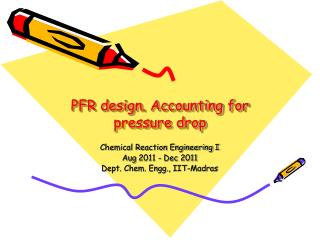DownloadDownload PresentationPFR design. Accounting for pressure drop

# PFR design. Accounting for pressure drop

Télécharger la présentation## PFR design. Accounting for pressure drop

- - - - - - - - - - - - - - - - - - - - - - - - - - - E N D - - - - - - - - - - - - - - - - - - - - - - - - - - -
##### Presentation Transcript

1. PFR design. Accounting for pressure drop Chemical Reaction Engineering I Aug 2011 - Dec 2011 Dept. Chem. Engg., IIT-Madras

2. Overview • Notation • PFR design equation (mass balance) • Pressure drop equation • Accounts for change in number of moles (due to reaction) • Does not consider phase change • Methodology, with examples • Liquid phase reaction: Calculations are simple • Gas phase reaction: Calculations are more involved

3. Notation • Usual notation applies. • FA = molar flow rate of A, FT = total molar flow rate • V = volume of reactor, Q = volumetric flow rate • D = diameter and A = cross-sectional area of PFR, L = length of PFR, z = distance (between 0 and L) • T = temperature, R = universal gas constant, P = pressure, PA = partial pressure of A • x = conversion, e = fractional increase in number of moles for 100% conversion, for a given feed conditions. • k = rate constant, CA = concentration of A • r = density, m = viscosity • ff = friction factor, Re = Reynolds number • <Vav> = average velocity of the fluid in the PFR. Used sparingly. • Subscript “in” indicates inlet. E.g. FA-in is molar flow rate of A at the inlet.

4. Definitions and formulas The following definitions are of use here: Definition of ‘x’ Definition of ‘e’ Definition of CA For gas phase only: From ideal gas law At constant temperature

5. Formulas For liquid as well as gas phase You don’t need to memorize these formulas

6. PFR design equation • Steady state conditions • For a first order reaction • At constant temperature

7. PFR design equation • For any other order of reaction also, write the equation such that dx/dz = f(x,P). i.e. The only unknowns on the RHS must be x and P • i.e. RHS must not have Q or CA. These are not known yet. • But Qin and CA-in are known and hence RHS may have these terms. • For an ‘n’th order reaction

8. Pressure Drop Equation • Under steady state conditions, Reynolds number is a constant • Even though local velocity changes • This is because density also changes with location • We assume that the viscosity of the medium remains the same, even when the reaction occurs • Under steady state conditions, (rQ) = constant • Using Re, Calculate the friction factor ff. • Write pressure drop equation

9. Solution • Solve both equations simultaneously • Initial conditions: • At z =0, P = Pin • At z = 0, x = 0 • Special case: • When e =0, solve the first equation and find ‘P’. Then substitute for ‘P’ in the second equation and solve for ‘x’

10. Example • Consider a gas phase reaction under isothermal conditions. (Isomerization) • A B • Pin = 10 atm, Q = 0.005 m3/s, T = 300 K, Pure A is fed, k = 0.1 lit/s, Molecular weight M = 60 g/gmol, Viscosity of the gas = 10-5 Pa-s • What should be the length of the PFR if it is constructed (a) using a 2 cm pipe and the conversion desired is 10%? (b) using a 1.5 cm pipe and the conversion desired is 10% and (c) using a 1.5 cm pipe and the conversion desired is 20%

11. Solution • e= 0. This simplifies the equations R=0.08206 atm-lit/(gmol-K)

12. Dia = 2 cm

13. Dia = 2 cm, expanded

14. Dia = 1.5 cm • 10% conversion is possible, but 20% is not

15. 2nd order reaction, k = 0.01 • Coupled equations give correct answer